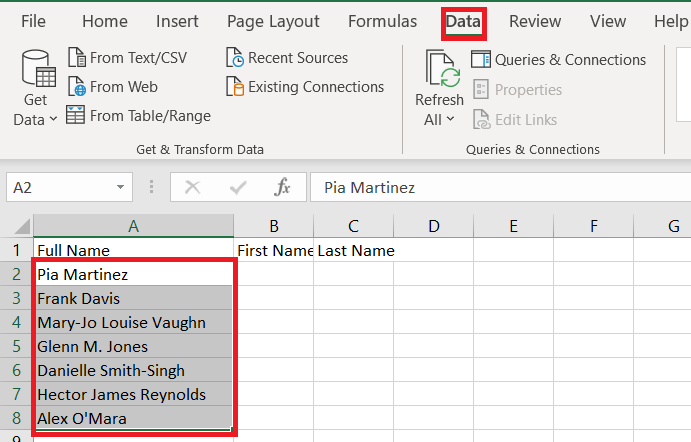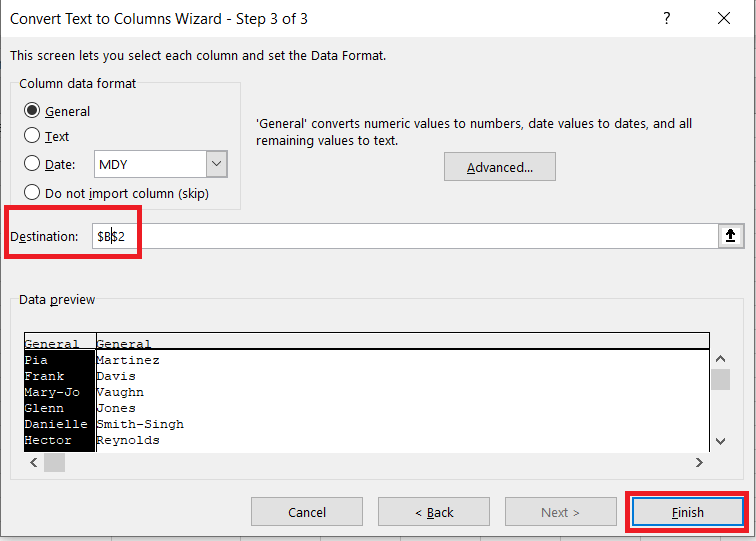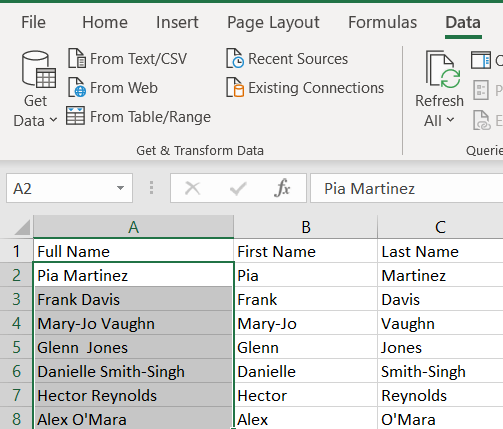# How To Separate First and Last Name in Excel

You can use Excel to break information into smaller pieces. Finding the data you need and manipulating it is an important goal for many Excel users.If you have a person’s full name, you may need to zero in on just their first name or their last name. For example, if you’re sending a friendly automated email to your clients, you need to use their first names to avoid sounding impersonal. If you’re looking over a list of poll respondents, it might be important to just use their last names, or to hide their last names to maintain anonymity.

Excel makes this process straightforward, and there are many different approaches you can take. Here is a tutorial that will help you create separate First Name and Last Name columns using formulas. We cover the issue of middle names as well.

## Excel Formulas for Splitting Names into Parts

Where do you start?

### Separating First Names

This is the generic formula:

=LEFT(cell,FIND(” “,cell,1)-1)

To execute it, replace cell with the cell pointer that contains the first full name that you want to split up. In this example, you want to select B2 and enter the formula:

`=LEFT(A2,FIND(" ",A2,1)-1)`

However, it’s important to note that on some devices, this formula uses semicolons instead of commas. So if the above formula doesn’t work for you, you might need to use the following version instead:

=LEFT(cell;FIND(” “;cell;1)-1)

In the example, you’d use:

`=LEFT(A2,FIND(" ",A2,1)-1)`

Now you can simply drag the fill handle down to the end of the First Name column.

The LEFT function lets you separate a string, starting from the left end of the text. The FIND part of this formula locates the first space in the full name, so you get the part of your full name that comes before an empty space.

Hence, hyphenated first names stay together, and so do first names that contain special characters. But your Full Name column won’t contain middle names or middle initials.

### Comma or Semicolon?

Why isn’t the formula the same for everybody?

For many Excel users, Excel functions use commas to separate input data. But on some devices, the regional settings are different.

To discover which symbol is used by your Excel, simply start typing in the formula. When you start enter =LEFT(, you will see a hover text that will suggest the right formatting.

### Separating Last Names

Take the same approach to separating last names. This time, you should use the RIGHT formula, which separates strings starting from the right side.

The formula you need is:

=RIGHT(cell, LEN(cell) – SEARCH(“#”, SUBSTITUTE(cell,” “, “#”, LEN(cell) – LEN(SUBSTITUTE(cell, ” “, “”)))))

In the example above, you would use the following formula in cell C2:

`=RIGHT(A2,LEN(A2)-SEARCH("#",SUBSTITUTE(A2," ","#",LEN(A2)-LEN(SUBSTITUTE(A2," ","")))))`

Once again, you may need to switch from the comma to a semicolon, meaning that you may need to use:

`=RIGHT(A2;LEN(A2)-SEARCH("#";SUBSTITUTE(A2;" ";"#";LEN(A2)-LEN(SUBSTITUTE(A2;" ";"")))))`

Hyphenated last names and last names with special characters stay intact.

Why is this formula more complex than the one for first names? It’s more difficult to separate middle names and middle initials out of last names.

If you want the middle names and initials to be listed with the last names, you can use the formula:

=RIGHT(cell, LEN(cell) – SEARCH(” “, cell))

or:

`=RIGHT(A2,LEN(A2)-SEARCH(" ",A2))`

or:

`=RIGHT(A2;LEN(A2)-SEARCH(" ";A2))`

But what if you want to separate the middle names? This is less common but it can be useful to know.

### Separating Middle Names

The formula for middle names is the following:

=MID(cell, SEARCH(” “, cell) + 1, SEARCH(” “, cell, SEARCH(” “, cell)+1) – SEARCH(” “, cell)-1)

In the example above, you get:

`=MID(A2,SEARCH(" ",A2)+1,SEARCH(" ",A2,SEARCH(" ",A2)+1)-SEARCH(" ",A2)-1)`

If your Excel uses semicolons, the formula is:

`=MID(A2;SEARCH(" ";A2)+1;SEARCH(" ";A2;SEARCH(" ";A2)+1)-SEARCH(" ";A2)-1)`

After entering the formula, drag the fill handle down. Here is a Middle Name column added to the example above:

If the full name contains no middle name or initial, your get zero-values in this column, which may be displayed as #VALUE!. To get blank cells in place of #VALUE!, you can use the IFERROR function.

=IFERROR(MID(cell, SEARCH(” “, cell) + 1, SEARCH(” “, cell, SEARCH(” “, cell)+1) – SEARCH(” “, cell)-1),””)

or:

`=IFERROR(MID(A2,SEARCH(" ",A2)+1,SEARCH(" ",A2,SEARCH(" ",A2)+1)-SEARCH(" ",A2)-1),"")`

or:

`=IFERROR(MID(A2;SEARCH(" ";A2)+1;SEARCH(" ";A2;SEARCH(" ";A2)+1)-SEARCH(" ";A2)-1);"")`

### One Approach to Separating Multiple Middle Names

What happens if someone on your list has multiple middle names? Using the above formula, only their first middle name will be retrieved.

To solve this issue, you can try a different approach to separating middle names. If you have the first name and last name columns created, you can simply cut them off. Everything that’s left will count as the middle name.

This formula is:

=TRIM(MID(cell1,LEN(cell2)+1,LEN(cell1)-LEN(cell2&cell3)))

Here, cell1 refers to the cell pointer under column Full Name, cell2 refers to the cell pointer under column First Name, while cell3 refers to the cell pointer under column Last Name. In the example above, we get:

`=TRIM(MID(A2,LEN(B2)+1,LEN(A2)-LEN(B2&D2)))`

or:

`=TRIM(MID(A2;LEN(B2)+1;LEN(A2)-LEN(B2&D2)))`

If you go with this formula, you won’t have to worry about zero-values.

## Quick Recap

Here are the formulas that you can use for splitting full names into parts:

First names: =LEFT(cell,FIND(” “,cell,1)-1)

Last names: =RIGHT(cell, LEN(cell) – SEARCH(“#”, SUBSTITUTE(cell,” “, “#”, LEN(cell) – LEN(SUBSTITUTE(cell, ” “, “”)))))

Middle names: =IFERROR(MID(cell, SEARCH(” “, cell) + 1, SEARCH(” “, cell, SEARCH(” “, cell)+1) – SEARCH(” “, cell)-1),””)

Alternative formula for middle names: =TRIM(MID(cell1,LEN(cell2)+1,LEN(cell1)-LEN(cell2&cell3)))

## Separating First and Last Names Without Using Formulas

If you don’t feel like typing out a bunch of formulas that may get entered in wrong, then take advantage of Excel’s built-in Convert Text to Columns Wizard.

1. Make sure the Data tab is selected from the menu at the top and highlight the column you want to convert.2. Then, click on Text to Columns.3. Next, make sure Delimited is selected and click Next.
4. Now, select Space from the options and click Next.5. Then, change the Destination to “\$B\$2” and click Finish.The end result should look like this.## A Final Word

There are many other ways to solve this problem in Excel. If none of the available options do what you need, do some more research.

Using formulas is relatively simple and it doesn’t depend on the version of Excel you’re using. But unfortunately, you still may run into errors.

For example, if a person’s full name starts with their family name, it will get split apart the wrong way around. The formulas will also have trouble with last names that contain prefixes or suffixes, such as le Carré or van Gogh. If someone’s name ends in Jr., that will get listed as their last name.

However, there are modifications you can add to solve these issues as they appear. Working with formulas gives you the flexibility you need to tackle these complexities.

## 2 thoughts on “How To Separate First and Last Name in Excel”Ali says:
Hi. Using the formula that you have stated above, how can we make the name Mary-Jo be displayed as Mary-jo?